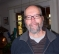## "Software Receiver Design" Timing Recovery QuestionStarted by 6 years ago5 replieslatest reply 2 years ago186 views
I got through the first 11 chapters of this book with no problems,
but I am now stuck on the beginning of Chapter 12, "Timing Recovery."
I'm attaching the page where I'm stuck (12.2, Example). I have spent
many, many hours trying to figure out the very last paragraph on this page,
but I am hopelessly confused.  The passage from the book describes
sampling a received signal x(t), with period T, and a Nyquist impulse
response which convolves the transmitter pulse, the channel response
(assumed to be identity), and the receiver filter into h(t). The
variable tau is the offset for the timing of the sampling. The impulse
response is simplified to the triangular shape in the figure. I think
I understand the second-last paragraph, when tau equals zero.  Where
I'm stuck is on the very last paragraph, which begins, "With tau = tau0 > 0,
the only two nonzero points among the sampled impulse response are at
h(tau0) and h(T + tau0), as illustrated in Figure 12.3." Why?  I think it
has to do with the sampling only being done at tau0 and T+tau0, but that's
just my guess.  And I don't get at all why h((k - i)T + tau0) equals
the quantities on the right, and why the quantities are divided by T.
If this question is already answered elsewhere, just point me to it.
Otherwise, I'll be eternally grateful for any illumination anyone can offer!
[ - ]I don't have a copy of that text, but the part you show looks very poorly worded, indeed.

Realize that 1/T is the magnitude of the slope of the two lines and the quantities make sense for what is shown, i.e., at tau0 the value is either tau0/T or 1-tau0/T if 0>tau0<1T.

The only two points in the graph that *aren't* non-zero are at t = 0 and t = 2T, so while the wording is tortured it is correct from the point of view that at tau0 = 0 the T-spaced samples at t = 0 and 2T equal zero, but not for any non-zero tau0.

Hope that helps a bit.   I agree that that wording is not at all clear.

[ - ]Thanks for the speedy reply!  So, your
explanation of the slope is a big help -- now I understand
the quantities on the right of the last line.  But I'm still
confused about the comment at the bottom of the page that "the
only two nonzero points among the sampled impulse response are
at h(tau0) and h(T+tau0)" since that doesn't match Figure 12.3
in the text, where h(t) is all nonzero between 0 and 2T --
is the idea that we only sample at t = tau0 and T+tau0, so of
course the values are zero when we don't sample?  Or am I just
missing something obvious?  Anyway, thanks, Slartibartfast,
you've helped me a lot.
[ - ]I think that's the part that is just badly worded if not downright wrong.   You're right that there are only two points that are zero, and they will only be sampled as zero when tau0 = 0.   I don't think you're missing anything.

[ - ]OK -- thanks again!

[ - ]